## InformationMathematics as we know it today is only a tool to symbolize quantities. Vortex-Based Mathematics (VBM) is different because it is a dynamic math that shows the relationships and thus the qualities of numbers rather than the quantities.

Members: 107
Latest Activity: Aug 31, 2019

## Vortex Based Mathematics ~ A second page available > Button under group membersMarko Rodin's Vortex Based Mathematics is a revolutionary system involving whole numbers, toroidal constructs and spirituality that explains the science of our Interdimensional Universe. Marko Rodin's discovery of Vortex Based Mathematics provides humanity with a unique and important key to the new interdimensional science we are seeking. The vortex takes you on a spiraling tour through the toroidal roller coaster of our interdimenstional universe. Please take time to enjoy these mind-opening videos ... from one of the planet's most "out-there" and inspiring talented people of our time - Marko Rodin.His understanding of maths and numbers is outstanding and the applications of his Vortex Based Mathematics to virtually anything imaginable ... from Free Energy to the physics of galaxies and potentially to the science of consciousness and spirituality ... represent a magnificent contribution to humanity. I concur with Marko’s postulate that the large scale structure of the Universe is a nested toroidal structure. The interdimensional aspects missing from current science to do with how the Universe really works will surely find a basis in the Marko Rodin's Vortex Mathematics.Through the introduction to Vortex Based Mathematics provided below, you will be able to see how energy is expressing itself mathematically. According to Marko, this math has no anomalies and shows the dimensional shape and function of the universe as being a toroid or donut-shaped black hole. This is the template for the universe and it is all within our base ten decimal system! You will enter a place where Numbers are Real and Alive not merely symbols for other things. You will discover that the relationships between numbers are not random or man-made but that numbers are actually elementary particles of which everything is composed. This lost knowledge was well known to our ancients and is now being uncovered for us today. Gradually you will come to see numbers in a simple yet profoundly perfect three-dimensional matrix grid pattern that forms the shape of a torus. The number grid reveals the calibration and timing for an engine that can take us throughout the universe and solve mankind's energy needs. Interested? Delve in. .. Want to find the answers to the unknown? Want to see a man-made black hole? Using a new math that shows that numbers are not man-made symbols, but are actually real, you will see and understand the answers to these questions. Also learn about:A particle that does not bend, but causes everything in the universe to curve around it.The three toroidal number patterns that are the basis to all mathematics (the pattern of numbers traversing the toroidal surface in, out, and around).The universe as an ecological system of renewal.Your body being made out of countless stars of the past.Proof of an omniscient supreme being.Making a coil that produces unlimited free energy.A number grid that shows the underpinning geometry of the universe.How everything is a torus.Making a propulsion source that can take you anywhere in the universe.Artificial Intelligence based on a binary triplet.The Aetheric Template in DNA that guides evolution.The repeating number pattern that solves pi and demonstrates it to be a whole number.A mathematics in which numbers do not move, but the functions move instead.How numbers form a 3D solid geometric object.The secret of a gyroscope -- why it vertically lifts itself upright.Wormholes, nested vertices, portals, and doorways.Why Dimensions are a result of how things move through space.The preferred frame of reference to the universe is based upon the fixed constant number 9.Number crunching and encription compression done through cross addition.The labrynth maze of the Doubling Circuits where they invert in the center.World boundary conditions and inversion seams of nested vortices.The bilateral symmetry of the decimal system resulting in polar number pairs having parity by being perfect mirrors of one another.The zero is always in the center in the form of the vortex hole.Multiplication series are unbroken rings from cross sections of the torus.A number cannot be created or destroyed and zero does not exist on a number line or in any multiplication series.The number 9 is the node and represents Spirit.The numbers 3 and 6 represent the Yin and Yang in the form of magnetism and the number 9 is the S curve.The base ten numerical counting system is triangulated.Infinity has an epicenter.The powers of ten occur by halfing.The torus skin models harmonic cascadence.( 1 divided by 7² or 1/49 = 0.020408163265306122448979591836735.

This 42 digit decimal applied to a circle show's a whole invisible tr3angulation which is a 9 fold dynamic with 7 star's of David, which numbers add up to 9)

thanks to Leben Nightmajic/Benjamin Gitchel for point this out...

**************************************************Mathematics as we know it today is only a tool to symbolize quantities. Vortex-Based Mathematics (VBM) is completely different because it is a dynamic math that shows the relationships and thus the qualities of numbers rather than the quantities.

Marko studied all the world's great religions. He decided to take The Most Great Name of Bahaullah (prophet of the Bahai Faith) which is Abha and convert it into numbers. He did this in an effort to discover the true precise mystical intonation of The Most Great Name of God. Since the Bahai sacred scripture was originally written in Persian and Arabic, Marko used the Abjad numerical notation system for this letter to number translation. This was a sacred system of allocating a unique numerical value to each letter of the 27 letters of the alphabet so that secret quantum mechanic physics could be encoded into words. What Marko discovered was that (A=1, b=2, h=5, a=1) = 9. The fact that The Most Great Name of God equaled 9 seemed very important to him as everything he had read in both the Bahai scriptures and other religious text spoke of nine being the omni-potent number. So next he drew out a circle with nine on top and 1 through 8 going around the circle clockwise. Then he discovered a very intriguing number system within this circle. Marko knew he had stumbled upon something very profound. This circle with its hidden number sequence was the "Symbol of Enlightenment." This is the MATHEMATICAL FINGERPRINT OF GOD.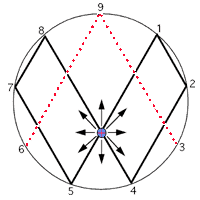Follow along as the amazing properties of this symbol unveil themselves to you. Put your pencil on number 1 and without picking up your pencil, move your pencil in a straight line to number 2, then 4, then across the center to 8. Notice that you are doubling. So next should be 16 and it is, but 1+6=7. So move your pencil to 7. Then 16 doubled is 32, but 3+2=5. So move your pencil to 5. Then 32 doubled is 64 and 6+4=10 and 1+0=1. And you're back to 1. So move the pencil across the center and back up to 1. The significance of the Mayan calender is that 64 is one complete cycle of infinity. Then it begins again with 64 doubled is 128 and 1+2+8=11, then 1+1=2. And so on. You will never get off this track as you keep doubling. Notice the infinity symbol has formed underneath your pencil, creating an ever-repeating pattern of 1, 2, 4, 8, 7, 5. Amazingly, this number sequence stays intact as you half numbers as well. Start again at the 1 but this time go backwards on the infinity symbol. Half of 1 is .5, so move your pencil to the 5. Then half of .5 is .25, and 2+5=7. So move your pencil to the 7. And half of .25 is .125 and 1+2+5=8. So move to the 8. Next half of .125 is .0625 and 0+6+2+5=13 and 1+3=4. So go across to the 4. And half of .0625 is .03125 and 0+3+1+2+5=11 and 1+1=2. So move to the 2. Forever staying on the route of 1,2,4,8,7,5 even backwards.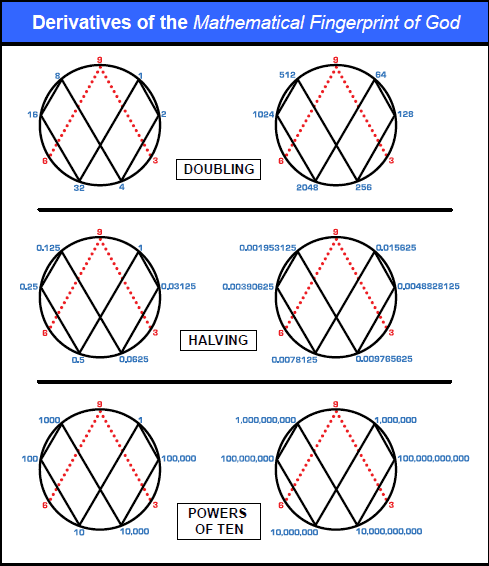As you replace the decimal numbers with their power (for example 0.5=10, .25=100, 0.125=1,000) you will see that the powers line up horizontally.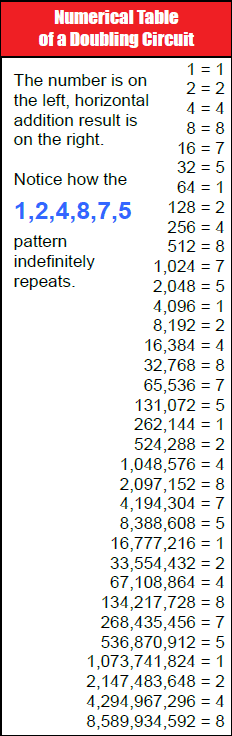At this point some of you might be thinking, "What in the world do these number patterns have to do with real world applications?" These number groupings piece together into a jig-saw-like puzzle pattern that perfectly demonstrates the way energy flows. Our base-ten decimal system is not man made, rather it is created by this flow of energy. Amazingly, after twenty years of working with this symbol and collaborating with engineers and scientists, Marko discovered that the 1,2,4,8,7,5 was a doubling circuit for a very efficient electrical coil.

There was still one more very important number pattern to be realized. On the MATHEMATICAL FINGERPRINT OF GOD notice how the 3, 9, and 6 are in red and do not connect at the base. That is because it is a vector. The 1,2,4,8,7,5 is the third dimension while the oscillation between the 3 and 6 demonstrates the fourth dimension, which is the higher dimensional magnetic field of an electrical coil. The 3, 9, and 6 always occur together with the 9 as the control. In fact, the Yin/Yang is not a duality but rather a trinary. This is because the 3 and 6 represent each side of the Yin/Yang and the 9 is the "S" curve between them. Everything is based on thirds. We think that the universe is based on dualities because we see the effects not the cause.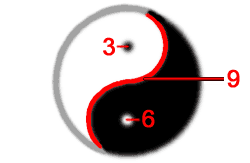When I say the oscillation between the 3 and 6 what I mean is that 3 doubled is 6 and 6 doubled is 12, but 1+2=3, and then 12 doubled is 24, but 2+4=6 and 24 doubled is 48, but 4+8=12 and 1+2=3. So the 3 and 6 go back and forth in their own separate dimension. This creates the other important pattern of (3,9,6,6,9,3,3,9).

Now lay out the number patterns on the 2D grid. Place the 3,9,6,6,9,3,3,9 number pattern in the center of the 1,2,4,8,7,5 number pattern going forward and the 1,2,4,8,7,5 number pattern going backwards this continuation creates an amazingly perfect number map. The MATHEMATICAL FINGERPRINT OF GOD is a legend or blueprint for the torus and can be visualized in a 2D form below.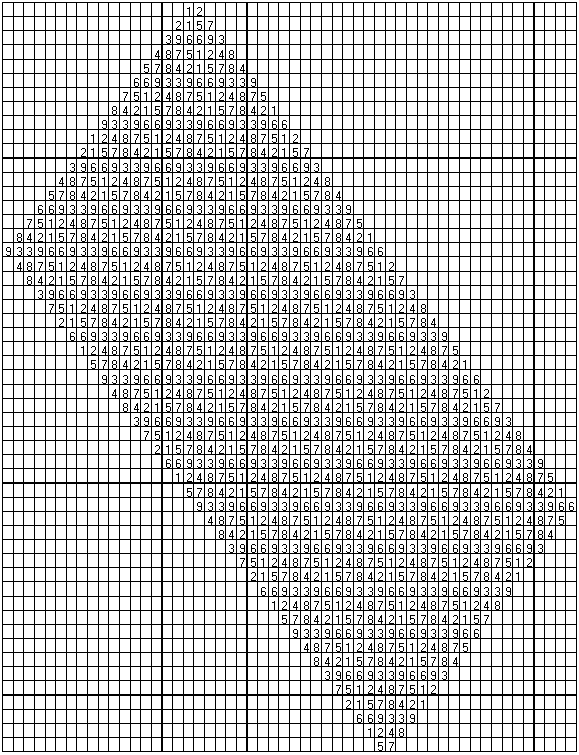The 2D number map forms the 3D skin of the torus and thus demonstrates the concept of the winding of the coil.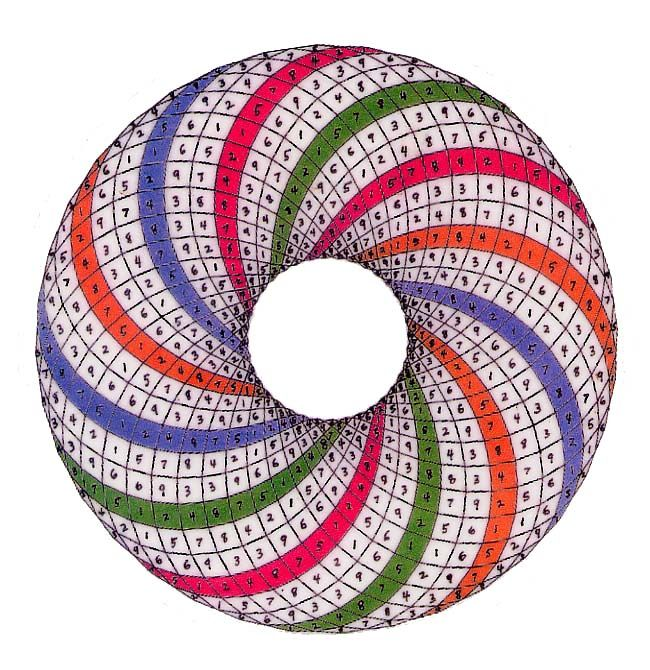And the 9 demonstrates the omni dimension which is the higher dimensional flux emanation called Spirit that always occurs within the center of the magnetic field lines. The last number left to be explained from The MATHEMATICAL FINGERPRINT OF GOD is the number 9. The number nine is Energy being manifested in a single moment event of occurrence in our physical world of creation. It is unique because it is the focal center by being the only number identifying with the vertical upright axis. It is the singularity or the Primal Point of Unity. The number nine never changes and is linear. For example all multiples of 9 equal 9. 9x1=9, 9x2=18, but 1+8=9, 9x3=27, but 2+7=9. This is because it is emanating in a straight line from the center of mass out of the nucleus of every atom, and from out of the singularity of a black hole. It is complete, revealing perfection, and has no parity because it always equals itself. The number nine is the missing particle in the universe known as Dark Matter.

The number nine lines up with the center of the infinity symbol and it is from this center that the linear emanations we call Spirit emanate from the center of mass outwards. Spirit is the only thing in the universe that moves in a straight line. Spirit is the inertia aether that Einstein postulated. Spirit is what makes everything else warp and curve around it. The perfect number patterns are actually created by this Spirit energy. Without Spirit the universe would become destitute and void. Spirit flow is the source of all movement as well as the source of the non-decaying spin of the electron.Numbers have temporal, spatial and volumetric qualities. For example, physics is the base ten number system. In other words numbers have bilateral or mirror symmetry and line up in stratified layers of horizontal planes. This is the same as physics' parity. There are nine simple multiplication series (one series for each discrete number 1-9) in Vortex-Based Mathematics. These multiplication series are based upon reducing all products to single digits via horizontal addition forming meaningful number sequences.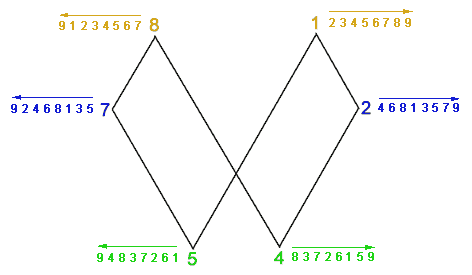There are three polar number pairs; 1 and 8, 2 and 7, 4 and 5. For example, multiples of 4 are 1x4=4, 2x4=8, 3x4=12 but 1+2=3, 4x4=16 but 1+6=7, 5x4=20 but 2+0=2, 6x4=24 but 2+4=6, 7x4=28 but 2+8=10 and 1+0=1, 8x4=32 but 3+2=5, 9x4=36 but 3+6=9. So multiples of 4 equal 4,8,3,7,2,6,1,5 and multiples of 5, the polar opposite of 4, are (5,1,6,2,7,3,8,4). Each pair forms number sequences that are identical but in opposing directions. So if you take the multiples of 5 and look at them running backwards they are the same as the multiples of 4 running forwards. In exactly the same way the multiplication series for the polar pair [1,8] is: (1,2,3,4,5,6,7,8) and (8,7,6,5,4,3,2,1) for the number 1 and the number 8 respectively. The multiplication series for the polar pair [2,7] is: (2,4,6,8,1,3,5,7) and (7,5,3,1,8,6,4,2). Everything is starting from the center outwards, from the singularity.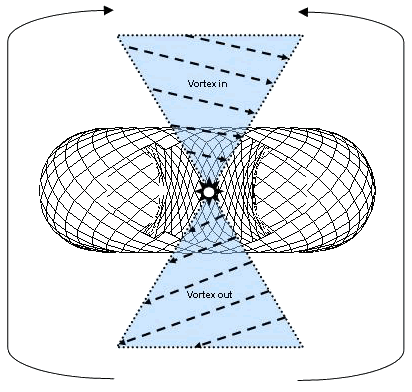Nature is expressing herself with numbers. The symmetry of our decimal system is a principle of nature. The 9 axis causes the doubling circuit and it is the point towards which matter converges and away from which it diverges or expands. Thus the polar number pairs will be mirror images of each other, both flowing in opposite directions from the central axis. There is perfect symmetry wrapped around a single point coiling outwards the way that petals are wrapped in a rose, or a nautilus shell spirals outward.

## Discussion Forum

### Human Vortex Quantom Math

Started by Seidr Aug 15, 2017.

We are light and energy.The etheric body coined by Madame Blavatskyhttps://en.wikipedia.org/wiki/Etheric_bodyWe are also electric.Nerve impulses in the brain and nervous system…Continue

### Vortex Based Math video's : presentation/ interview

Started by Jolan ☯ May 29, 2013.

Free Energy| Rodin Coil Vortex Based Math | TEDxCharlotte 2010 Randy Powell Red Ice Radio - Randy Powell - Hour 1 - Vortex Based Mathematics & The ABHA Tor Gator showing the mechanics of his 18 x 18 grid drawn out geometrically. …Continue

### Oddity: How I discovered Marko Rodin via English gematria

Started by Gary Val Tenuta. Last reply by Jolan ☯ Apr 25, 2013.

In the intro to this page there is a list of things that can be learned from watching Marko Rodin's video. Number 18 on the list says:The preferred frame of reference to the universe is based upon the fixed constant number 9.What I'm going to…Continue

Tags: 117, sacred, 144, 594, bible

Started by Robert Searle Jul 5, 2012.

My evolving project may have some relevance.http://www.p2pfoundation.net/Multi-Dimensional_ScienceContinue

### Abha Torus 8-1 9x9 Nested Vortices Explanation (provisional)

Started by Sunmover. Last reply by Dean Marston Feb 22, 2012.

An attempt to visualise and understand the allocation of numbers to and the activation of the Nested Vortices Circuits. There are many minor errors although the principles at its core stand true - this is a test film giving me the opportunity to…Continue

### Rodin Coil and the Masons

Started by ☤Tris☤. Last reply by ॐBlessedॐ Sep 25, 2011.

Maybe this is a true correlation? maybe not? You be the judge. Continue

Tags: masons, the, and, coil, rodin

### Building a Tesla coil from 120v to 12000 volts blueprint

Started by ॐBlessedॐ. Last reply by ॐBlessedॐ Sep 25, 2011.

here is the PDF

## Comment Wall

CommentAlchemist
Comment by Will Bierre on September 6, 2013 at 11:01am

I think group members might find my blog post interesting:

Tori, FoL and Consciousness - Part 1Mystic
Comment by Jolan ☯ on August 28, 2013 at 7:26amMystic
Comment by Jolan ☯ on August 28, 2013 at 7:19amMystic
Comment by Jolan ☯ on May 29, 2013 at 9:07am

Free Energy| Rodin Coil Vortex Based Math | TEDxCharlotte 2010 Randy Powell

Red Ice Radio - Randy Powell - Hour 1 - Vortex Based Mathematics & The ABHA Tor

Gator showing the mechanics of his 18 x 18 grid drawn out geometrically.

http://www.americanantigravity.com - Marko Rodin. the inventor of vortex mathematics, describes his two presentations at the TeslaTech 2012 Conference. He briefly discusses the applications of a colleague's work to artificial intelligence as well as extensively describing the applications of vortex mathematics across a variety of disciplines.

Marko Rodin's Vortex Mathematics is a scientific and numerical system involving whole numbers, toroidal constructs and spirituality that he claims describes underlying principles at the foundation of science and physics. Rodin describes Vortex Mathematics as the means by which energy expresses itself mathematically, in a manner without anomalies that describes the dimensional shape and function of the universe as being a toroidally-shaped black holeMystic
Comment by Jolan ☯ on April 22, 2013 at 8:09amMystic
Comment by Jolan ☯ on April 22, 2013 at 8:08amMystic
Comment by Jolan ☯ on April 16, 2013 at 2:14am

Awesome, ty Mark ;)Comment by Mark Salot on April 2, 2013 at 1:44amMystic
Comment by Jolan ☯ on December 27, 2012 at 5:21amMystic
Comment by Jolan ☯ on December 25, 2012 at 5:37am